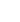15min:
PRECISION MEASUREMENT OF THE IONIZATION ENERGY OF THE GK~1g+~(v=1,N=1) STATE OF MOLECULAR HYDROGEN..

M. BEYER, D. SPRECHER AND F. MERKT, ETH Zürich, Laboratorium für Physikalische Chemie, Wolfgang-Pauli-Strasse 10, 8093 Zürich, Switzerland.

The ionization energy of the GK~1g+~(v=1,N=1) state of ortho H2 has been determined at a precision of 1.2~MHz by near-infrared laser spectroscopy.

The measurement was performed by first exciting molecular hydrogen from the X~1g+~(v=0,N=1) state to the GK~1g+~(v=1,N=1) state in a resonant two-photon process via the B~1u+~(v=3,N=2) state and then measuring the frequency of the transition between the GK~1g+~(v=1,N=1) state and the 56p~(S=0,N=1) Rydberg state belonging to the series converging on the X+~2g+~(v+=0,N+=1) ground state of ortho H2+. The ionization energy of the GK~1g+~(v=1,N=1) state was obtained by adding this frequency to the binding energy of the 56p~(S=0,N=1) Rydberg state which has been determined previously by millimeter-wave spectroscopy and multichannel quantum-defect theory .

For the measurement we used a homebuilt pulsed NIR laser with Fourier-transform-limited linewidth and adjustable pulse duration. To reach the desired accuracy, systematic errors originating from ac and dc Stark shifts, from pressure shifts, and from the frequency shifts and chirps accompanying the generation of the NIR laser pulses were quantified. The ionization energy of the GK~1g+~(v=1,N=1) state will be compared with earlier results .

New attempts of measuring the binding energy of the EF~1g+ state will also be mentioned.